SSAT Upper Level Math : Data Analysis / Probablility

Example Questions

Example Question #41 : Data Analysis / Probablility

Jeff collects basketball cards of players on his three favorite teams. He decides to put 5 cards from each team in a paper bag and then to draw out 3 cards at random. What are the odds of him getting one player from each team?

50%

19.8%

6.6%

33%

27.5%

27.5%

Explanation:

For this problem, we will multiply together the odds of each draw (assuming he draws one card at a time...the odds won't change if he draws three at once, but it's easier to visualize this way) resulting in a card that works for Jeff's goal of having one player from each team. The first draw cannot fail, as he needs one player from each team and the first card he draws must be from one of the teams. After this draw, he has 14 cards remaining, and 10 of these are players on the two teams that can still offer a player.

So the odds of a successful second draw are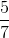.

The last draw is the trickiest, as there would now be 13 cards remaining, with only 5 being players from the team that he still needs represented. When we multiply all of these odds together, we get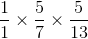which is 27.5%.

Example Question #44 : Probability

Presented with a deck of fifty-two cards (no jokers), what is the probability of drawing either a face card or a spade?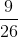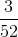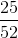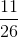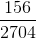Explanation:

A face card constitutes a Jack, Queen, or King, and there are twelve in a deck, so the probability of drawing a face card is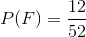.

There are thirteen spades in the deck, so the probability of drawing a spade is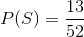.

Keep in mind that there are also three cards that fit into both categories: the Jack, Queen, and King of Spades; the probability of drawing one is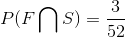Thus the probability of drawing a face card or a spade is: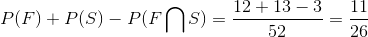Example Question #48 : Probability

Set A: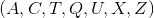Set B: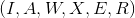One letter is picked from Set A and Set B. What is the probability of picking two consonants?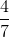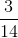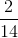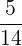Explanation:

Set A:Set B:In Set A, there are five consonants out of a total of seven letters, so the probability of drawing a consonant from Set A is.

In Set B, there are three consonants out of a total of six letters, so the probability of drawing a consonant from Set B is.

The question asks for the probability of drawing two consonants, meaning the probability of drawing a constant from Set A and Set B, so probability of the intersection of the two events is the product of the two probabilities: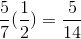Example Question #49 : Probability

Set A:Set B:One letter is picked from Set A and Set B. What is the probability of picking at least one consonant?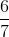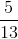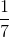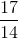Explanation:

Set A:Set B:In Set A, there are five consonants out of a total of seven letters, so the probability of drawing a consonant from Set A is.

In Set B, there are three consonants out of a total of six letters, so the probability of drawing a consonant from Set B is.

The question asks for the probability of drawing at least one consonant, which can be interpreted as a union of events. To calculate the probability of a union, sum the probability of each event and subtract the intersection: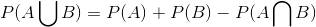The interesection is: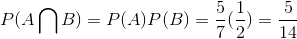So, we can find the probability of drawing at least one consonant: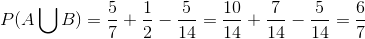Example Question #50 : Probability

Set A:Set B:One letter is drawn from Set A, and one from Set B. What is the probability of drawing a matching pair of letters?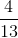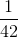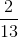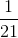Explanation:

Set A:Set B:Between Set A and Set B, there are two potential matching pairs of letters: AA and XX. The amount of possible combinations is the number of values in Set A, multiplied by the number of values in Set B,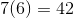.

Therefore, the probability of drawing a matching set is: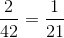Example Question #51 : How To Find The Probability Of An Outcome

In a particular high school, 200 students are freshmen, 150 students are sophomores, 250 students are juniors, and 100 students are seniors. Twenty percent of freshmen are in honors classes, ten percent of sophomores are in honors classes, twelve percent of juniors are in honors classes, and thirty percent of seniors are in honors classes.

If a student is chosen at random, what is the probability that that student will be a student who attends honors classes?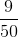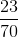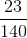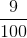Explanation:

First calculate the number of students: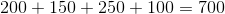The probability of drawing an honors student will then be the total number of honors students divided by the total number of students attending the school: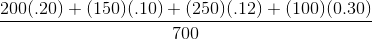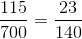Example Question #51 : Probability

In a particular high school, 200 students are freshmen, 150 students are sophomores, 250 students are juniors, and 100 students are seniors. Twenty percent of freshmen are in honors classes, ten percent of sophomores are in honors classes, twelve percent of juniors are in honors classes, and thirty percent of seniors are in honors classes.

If a student is chosen at random, what is the probability that that student will be a senior student and a student who does not attend honors classes?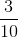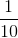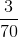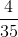Explanation:

First calculate the number of students:The percentage of seniors that do not attend honors classes is: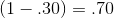Therefore, the probability of selecting a student who is a senior and one who does not attend honors classes is: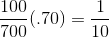Example Question #42 : Data Analysis / Probablility

Toss a coin twice.  Roll a six sided dice once.  What is the probability of flipping two heads and rolling a one?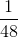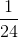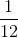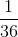Explanation:

Each toss and roll of the dice are independent events.  The probability of rolling either a head or tail on the first and second trial is one-half.

The probability of rolling any number on the dice issince there is one number on each of the six faces of the dice.

Multiply all the probabilities.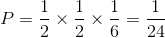Example Question #43 : Data Analysis / Probablility

A standard deck of 53 cards (including the joker) is altered by adding the joker and the aces from another deck. What are the odds against a randomly drawn card being an ace?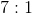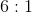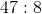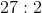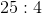Explanation:

Adding the joker and the four aces to a 53-card deck will yield a deck of 58 cards, which contains 8 aces and 50 other cards. The odds against drawing an ace at random are therefore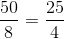- that is, 25 to 4.

Example Question #44 : Data Analysis / Probablility

A standard deck of 52 cards is altered by adding the hearts from another deck. What are the odds against a randomly drawn card being a jack?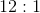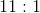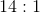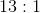None of the other responses give the correct odds.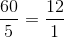- that is, 12 to 1, just as in the unaltered deck.